This page describes how costs are calculated for individual converters in the model. The calculations are designed to be standardised so that they can be used for several types of plants (electricity, heat, hydrogen etc.). These calculations are done for each converter.

This page gives an overview of all the components of the cost calculations.

## Total costs#

The total costs define the yearly total costs of a technology in euro's. The total costs are split up in the fixed and variable costs of the converter. It therefore adds up Fixed costs and Variable costs (see below). The total costs are a combination of cost of capital, depreciation, and fixed operation and maintenance costs.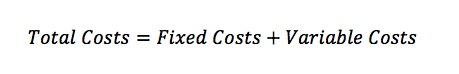## Fixed costs#

The fixed costs are the costs that have to be paid yearly, independent of the full load hours of the plant. When more plants are installed, the fixed costs will go up, but when only the number of full load hours changes, these costs remain the same.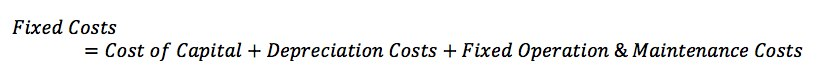#### Cost of capital#

The cost of capital is the amount of money that is spent yearly to finance the required investment for the plant. It assumes that the capital required either costs money to finance, or could have been used to generate money elsewhere. This is a percentage of the average investment over the lifetime of a plant, which is called the WACC, the weighted average cost of capital. During the construction of the plant capital costs must also be paid and this is factored into the costs by taking into account the construction time into the equation. See the WACC section for more information.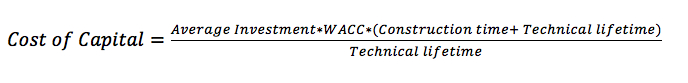Where:

• Average investment = (initial investment + initial investment for Carbon Capture and Storage (CCS) + installing costs + Decommissioning Costs ) / 2
• WACC = Weighted average cost of capital
• Construction Time = the time it takes to construct a typical plant of this type
• Technical Lifetime = the average amount of time that a plant can continue operating

#### Depreciation Costs#

Next to the cost of capital, a plant will decrease in value every year. Using the straight line depreciation method the yearly depreciation is calculated.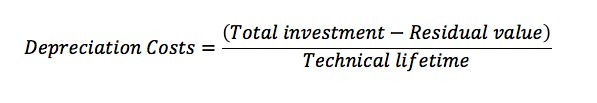Where:

• Total investment = the initial investment + initial investment for Carbon Capture and Storage (CCS) + installing costs + Decommissioning Costs
• Residual Value = the remaining value after a plant is retired (for example: selling raw material, reclaimed land, etc)
• Technical lifetime = the average amount of time that a plant can continue operating

#### Fixed Operation and Maintenance costs#

Operation and Maintenance costs can have both a fixed and a variable part. The fixed part is the costs that are made yearly, independent of whether the plant is used or not. Examples of fixed costs are the costs for personnel that have to do yearly maintenance such as cleaning the plant. Fixed O&M Costs are specified per year and found directly from research. This means that Fixed O&M Costs have no calculations associated with them.

## Variable costs#

The variable costs depend on how much the available plants are used. It is a combination of fuel costs, the variable part of operation and maintenance costs, and if applicable, CO2 emission costs.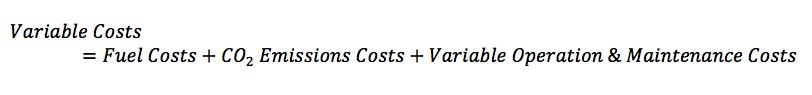#### Fuel costs#

Here, the fuel costs for the plant are calculated, based on the efficiency of the plant. The cost of the energy carrier that is consumed is used, except for electricity and steam/hot water. The production of these energy carriers already have costs assigned, so these would be counted double if they would be calculated here again.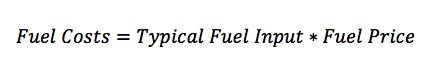Where:

• Typical fuel input = the yearly input of fuel for a typical plant of this type
• Fuel price = the fuel price of the type(s) used by this plant for the year of calculation

#### CO2 emission costs#

Plants in certain sectors need to have emission rights for the CO2 they emit. If they do, the amount of CO2 emitted is calculated based on the carbon content of the energy carrier used, and whether part of the CO2 is captured or not. The costs for CO2-emission is calculated by multiplying the emitted amount of CO2 with the cost of CO2-emission rights in the area and the percentage of CO2-rights that has to be paid.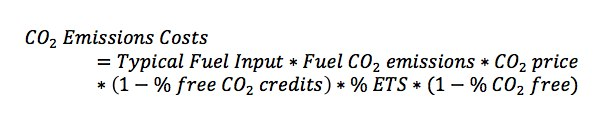Where:

• Typical fuel input = the yearly input of fuel for a typical plant of this type
• Fuel CO2 emissions = the typical amount of CO2 emitted when burning this fuel
• CO2 price = the price of emitting one unit of CO2
• % free CO2 credits = factor specifying how much of the CO2 credits are given away for free in the current country (or area)
• % ETS = factor specifying which part of this plant falls under the European Emissions Trading scheme
• % CO2 free = factor specifying how much of these CO2 emissions do not have costs associated with them (example: non-energetic emissions of CO2)

#### Variable Operation and Maintenance costs#

Here the variable operation and maintenance costs are calculated. These for example include costs for additional cleaning and service costs that depend of the number of full load hours of the plant.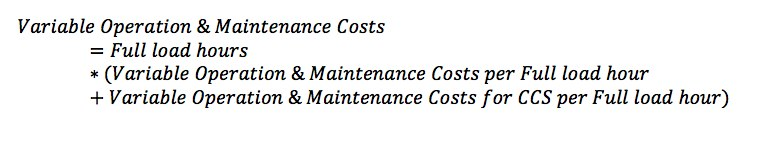Where:

• Full load hours = the typical yearly full load hours of a plant of this type
• Variable Operation & Maintenance Costs per Full load hour = the normal costs for operating and maintaining the plant for one full load hour
• Variable Operation & Maintenance Costs for CCS per Full load hour = the additional costs for operating and maintaining the Carbon Capture and Storage (CCS) part of a plant for one full load hour